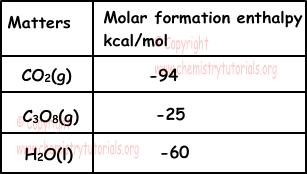EN | ES | DE | NL | RU

Thermochemistry Exam1 and Problem Solutions

Thermochemistry Exam1 and  Problem Solutions

1. Which ones of the following reactions are endothermic in other words ∆H is positive?

I. H2O(l) + 10,5kcal → H2O(g)     ∆H1

II. 2NH3 +22kcal →N2 + 3H2 ∆H2

III. Na + Energy → Na+1 + e-     ∆H3

Solution:

When matters change state from liquid to gas, they absorb energy. I is endothermic reaction. ∆H1 is positive.

In decomposition reactions energy (heat) is absorbed. III is endothermic reaction. ∆H2 is positive.

To remove one electron from atom we should give energy, so III is endothermic reaction and  ∆H3 is positive.

2. Given table shows  standard molar enthalpy of formation of some matters.Find enthalpy of C3H8(g) + 5O2(g) → 3CO2(g) + 4H2O(l) using data given in the table below.

Solution:

C3H8(g) + 5O2(g) → 3CO2(g) + 4H2O(l)

∆H=[3∆HCO2 + 4∆HH2O] - [1∆HC3H8 + 5∆HO2]

Since O2 is element, molar formation enthalpy of it is zero.

∆H=[3.(-94) + 4.(-60)] - [1.(-25) + 5.0]

∆H=-522 + 25

∆H=-497 kcal/mol (it is negative, in other words reaction is exothermic)

3. To calculate enthalpy of ; CO2(g) + H2(g) → CO(g) + H2O(g) which ones of the following must be known?

I. Molar formation enthalpy of H2O(g)

II. Molar formation enthalpies of CO(g) and CO2(g)

III. Enthalpy of reaction; H2(g) + 1/2O2(g) → H2O(g)

Solution:

We find enthalpy of CO2(g) + H2(g) → CO(g) + H2O(g);

∆H=Σa∆H(F.(Products) - Σb∆H(F.(Reactants)

∆H=[∆HCO + ∆HH2O] - [∆HCO2 + ∆HH2]

Since H2 is element, molar formation enthalpy of it zero.

So, we must know I and II to find enthalpy of given reaction.

4. Find molar combustion enthalpy of C2H5OH using following molar enthalpies of matters;

∆H C2H5OH(l)= -67 kcal/mol

∆H CO2(g)= -94 kcal/mol

∆H H2O(l)= -68 kcal/mol

Solution:

We should first write combustion reaction of C2H5OH;

C2H5OH(l) + 3O2(g) → 2CO2(g) + 3H2O(s)

We use following formula to find unknown enthalpy;

∆HReaction=Σa∆H(Products) - Σb∆H(Reactants)

∆HCombustion=(2∆HCO2(g) + 3 ∆HH2O(l) ) - (∆HC2H5OH(l) + 3∆HO2)

∆HCombustion=[2.(-94) + 3.(-68)] - [-67]

∆HCombustion= -325 kcal/mol

5. There are 32 g S in 1000 g vitreous calorimeter having 1000 g water in it. If 32 g S is burned up in calorimeter, temperature rises from 20 0C to 90 0C. Find molar combustion enthalpy of S.

Solution:

We find heat gained by glass and water during combustion by formula;

Q=m.c.∆T

Qglass=1000.0,2.(90-20)=14000 cal

Qwater=1000.1.(90-20)=70000 cal

Qcalorimeter=70000 + 14000= 84000 cal

1 mol S is 32 g.

Molar combustion enthalpy of S is 84000 cal or 84 kcal.

Since it is combustion enthalpy;

∆HCombustionS= -84 kcal/mol

The Original Author: